American Journal of Modern Physics
Volume 4, Issue 6, November 2015, Pages: 267-273

Light Speed in Vacuum Is not a Constant and Time Doesn’t Change with Velocity – Discrepancies Between Relativities and Yangton & Yington Theory

Edward T. H. Wu

Solar Buster Corporation, Los Angeles, USA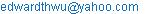Edward T. H. Wu. Light Speed in Vacuum Is not a Constant and Time Doesn’t Change with Velocity – Discrepancies Between Relativities and Yangton & Yington Theory. American Journal of Modern Physics. Vol. 4, No. 6, 2015, pp. 267-273. doi: 10.11648/j.ajmp.20150406.12

Abstract: Light Speed in vacuum, instead of being a constant, it changes with those observers moving at different speeds and directions with respect to light origins. Time, on the other hand, instead of moving slower with the traveler, it always keeps the same rate. These facts disagree with Einstein’s Special Relativity. Light speed in vacuum is a constant only if it is observed from light origins and those positions in stationary with light origins in Absolute Space System. This is because the emission of a Yangton and Yington circulation pair (Wu’s Particle or Still Photon) from the surface of a matter (String Structure or Higgs Boson) to form a free photon traveling in vacuum is a Non-Inertia Transformation and it only requires a small fixed amount of Force of Separation. Since light speed is not a constant to those observers moving at different speeds and directions with respect to light origins, Velocity Time Dilation derived from Einstein’s Special Relativity is not true and could never exist. Absolute Space System, Vision of Light and Non-Inertia Transformation are introduced to explain the relationships between Space, Time and Relativities. Doppler Effect, Blue Shift and Red shift are due to the Non-Inertia Transformation of light emission. Length contraction is caused by the difference of Visions of Light instead of Velocity Time Dilation. In an Inertia System, because of the same Visions of Light, the same light speeds in vacuum can be observed by all observers. Furthermore, Michelson - Morley Experiment proves that for two split light beams traveling in vacuum, the same light speeds can also be observed. Time is the measurement of the cycles of a fundamental process from start to end of an event. Both time and light speed at large gravitational field have relatively slower rates, which may be caused by the longer period and lower frequency of Yangton and Yington circulation due to the influence of large gravitational field. This agrees well with Gravitational Time Dilation in Einstein’s General Relativity.

Keywords: Special Relativity, Light Speed, Vision of Light, Velocity Time Dilation, Gravitational Time Dilation, Length Contraction, Michelson-Morley, Yangton, Yington, Photon, Wu’s Particle

Contents

1. Introduction

Yangton and Yington Theory (Ref. 1 & Ref. 2) has been proposed by Edward T. H. Wu successfully in explanations of the creation of Universe, unification of Four Forces, combination of Quantum Mechanics with Particle Physics, and the structures of Wu’s Particle (or Still Photon), Photon, Quarks, Higgs Boson, Electron, Neutron, Proton and other particles with String Theory, as well as their transformations.

In this paper, Yangton and Yington theory will be used to explain the nature of photon emission. Light speed in vacuum will also be thoroughly studied. In addition, with the concepts and definitions of Absolute Space System, Vision of Light and Non-Inertia Transformation introduced in this paper, Michelson - Morley Experiment, Doppler Effect, Red Shift, Length Contraction, Velocity Time Dilation and Special Relativity will also be discussed and analyzed for better understanding.

Finally, the meaning of Time will be explained, and Gravitational Time Dilation in General Relativity will be interpreted by the influence of gravitational field upon the period and frequency of Yangton and Yington circulation.

2. Absolute Space System

In the Universe, everything moves with respect to each other, there is no absolute reference. However, when a photon emitted from a light source, it generates a straight optical path from light origin (not light source) into vacuum space. Since there is no inertia, light origin has an absolute fixed position in the space which doesn’t move with light source neither Earth nor anything else. It is therefore proposed that an Absolute Space System can be formed by three perpendicular optical paths at light origin in the instance that the photon is emitted from the light source. Absolute Space System does exist in the Universe, but it cannot be visualized neither be duplicated after the first instance of photon emission.

3. Vision of Light

Observer’s vision can be constructed by superimposing observer’s positions in each time frame onto observer’s final position. Fig. 1 shows the schematic diagrams of the Visions of Light of an emitted photon with respect to observers at light origin, ground and light source in Absolute Space System, where ground and light source are drifted apart from light origin due to the motions of Earth (VE) and light source (VS). After a time interval ∆t, assuming all motions are at constant speeds, the Visions of Light of those observers can be presented by the following straight lines: AP – the Vision of Light observed by the observer at light origin (black line), BP – the Vision of Light observed by the observer at ground (red line) and CP – the Vision of Light observed by the observer at light source (green line) respectively. They are all ended at the final position of the emitted photon (point P).

Because Visions of Light are different to each observer moving at different speeds and directions with respect to light origin (Fig. 1), also the speed of light is calculated by the Vision of Light divided by the traveling time of light, therefore the light speeds observed by moving observers are different from each other by a ratio same as that of Visions of Light.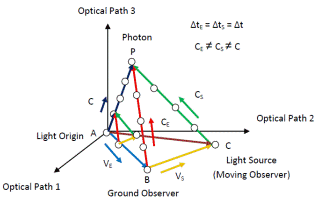Fig. 1. Visions of Light of an emitted photon observed by observers at light origin (black line), ground (red line) and light source (green line) in Absolute Space System.

4. Michelson - Morley Experiment

The purpose of Michelson - Morley Experiment is to detect the difference of light speeds caused by the motion of Earth through optical interference. Fig. 2 shows the Visions of Light of a beam of photons: AP is the Vision of Light observed at light origin (red line) which is stationary in Absolute Space System and BP is the Vision of Light observed at light source or ground (black line) which is drifted apart from light origin by Earth motion VE. When the photons reaches the semi-transparent mirror from Vision of Light observed at light source or ground BP, it splits into two perpendicular light beams and both get bounced back from the two end mirrors placed at equal distance from the center of the semi-transparent mirror. Two beams are then recombined at the semi-transparent mirror and finally received by the detector. Since light source or ground, semi transparent mirror, two end mirrors and detector are all in stationary with respect to Earth, and CE is the light speed observed by observers at light source or ground (stationary to Earth), also in alliance with the Vision of Light at Light origin (red line) in Absolute Space System, there is no additional influence from Earth motion needs to be considered in study of Vision of Light at light source or ground (black line). Because there is no optical interference, Michelson - Morley Experiment proves that for two split light beams traveling in vacuum, the same light speeds can be observed by those observers in the same Inertia System.

Furthermore, because of the circulation and rotation of Earth, the actual displacement of Earth AB instead of being a straight line, it is slightly curved, therefore, BP, PM and PD are also slightly curved. However, the two light beams received by the detector always have the same light speeds and no optical interference can be found in Michelson - Morley experiment.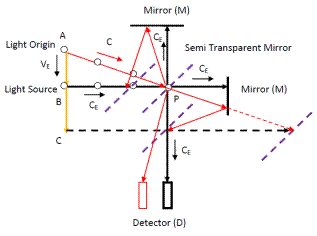Fig. 2. Michelson - Morley Experiment with the Visions of Light observed by the observers at light source or Earth (black line) and light origin (red line).

5. Light Speed

5.1. Absolute Light Speed

Because Yangton and Yington circulation pair known as Wu’s Particle or Still Photon (Ref. 1 & Ref. 2) has no mass, the separation of Wu’s Particle from the surface of a matter (String Structure or Higgs Boson) to form a free photon traveling in vacuum is a Non-Inertia Transformation. Also, Wu’s Particle is the finest building block of all matters; it requires a small fixed amount of Force of Separation (Fig. 3). Therefore, it is reasonable to assume that there is one and only constant Absolute Light Speed C in vacuum with a straight direction that can be observed by the observers at light origins and those in stationary with light origins in Absolute Space System.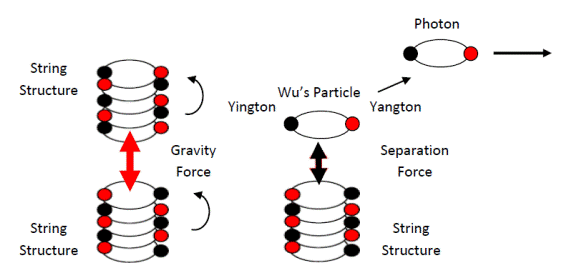Fig. 3. Gravity Force between two String Structures (Higgs Bosons) versus Force of Separation between Photon and String Structure (Higgs Boson).

5.2. Different Light Speeds

Because the speed of light is calculated by the Vision of Light divided by the traveling time of light. With different Visions of Light, it is obvious that different light speeds in vacuum can be observed by moving observers other than those at light origins and those in stationary with light origins in Absolute Space System. As shown in Fig. 1, in addition to the Absolute Light Speed C observed by the observer at light origin, light speed CE can be observed by the observer at moving ground (VE) and light speed CS can be observed by the observer at moving light source (VS). This is different from Einstein’s Special Relativity (Ref. 3) which claims that light speed in vacuum is always a constant no matter where the light sources and observers are.

5.3. Light Speed in Inertia System

As a photon traveling in vacuum with a given angle between the photon and the moving observer, same light speeds can be observed by all observers in the Inertia System of the moving observer. This phenomenon can be implied in two aspects:

1.  With respect to the observers – As shown in Fig. 4, the same Visions of Light of the photon traveling in vacuum with a given angle between the photon and the moving observer can be observed by all observers in the Inertia System of the moving observer.

2.  With respect to the light beam – Michelson - Morley Experiment proves that two split light beams have the same light speeds observed by the observers in the same Inertia System.Fig. 4. Vision of Light (blue line) observed at light origin and the Vision of Light (red line) observed at light source and those in stationary to light source.

5.4. Light Speeds on Earth

In reality, as shown in Fig. 1 the speed of Earth VE and the speed of the moving observer or light source on Earth VS are both extremely small comparing to the Absolute Light Speed C, therefore light speeds observed by ground observers, no matter in motion or at rest to Earth, have values very close to the Absolute Light Speed C which is about 3 x 108 m/sec. However, if an observer moves at a speed as fast as C in a parallel direction to light beam, then the light speed CS (Fig. 1) observed by the moving observer can be as small as zero which means the photon is idle or frozen with respect to the observer.

5.5. Limit of Light Speed

Because Wu’s Particle is the finest building block of all matters (Ref. 1 & Ref. 2), when it separates from the surface of a substance (String Structure or Higgs Boson) to form a free photon (Ref. 1), requires only a minimum of Force of Separation to generate the highest possible speed. Therefore, it is suggested that the Absolute Light Speed C (about 3 x 108 m/sec) is the limit of the highest speed of any substance in the Universe.

6. Gravitational Lensing

When a photon or a light beam passes through a gravitational field, just like that in the transparent material, light speed is reduced and direction is bending. This phenomenon is known as Gravitational Lensing.

7. Doppler Effect

The frequency of a wave-like signal such as sound or light depends on the movement of the sender and the receiver. This phenomenon is known as the Doppler Effect (Ref. 4)

When the source of light is moving toward the observer, each successive photon is emitted from a position closer to the previous photon. Therefore, the wavelength between two subsequent photons is smaller which causes an increase in the frequency or shift to blue end of the spectrum. This is commonly known as Blue Shift. Conversely, if the source of light is moving away from the observer, each photon is emitted from a position farther from the previous photon, resulting longer wavelength between two subsequent photons which causes a reduction in the frequency or shift towards red end of the spectrum. This is known as Red Shift (Fig. 5) (Ref. 5 & Ref. 6). Both Blue Shift and Red Shift phenomena can exist only if that the photon emission is a Non-Inertia Transformation process.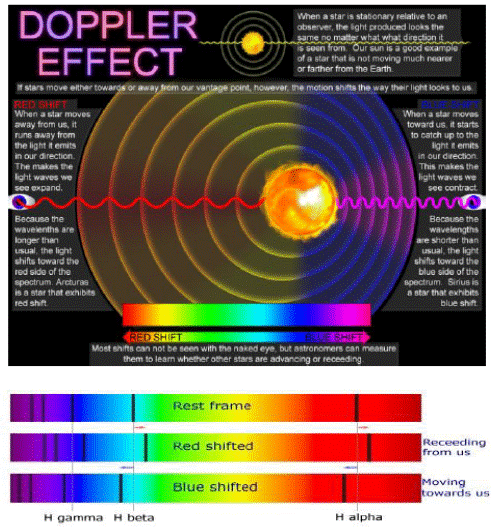Fig. 5. Doppler Effect and Blue Shift & Red Shift Phenomena.

Both blue shift and Red Shift phenomena can also be derived by mathematics as follows:

Zero Shift – Both light source (star) and observer are in stationary to each other, f is the frequency and λ is the wave length measured by the observer

C/f = λ

Blue Shift – Light source (star) moves towards observer at speed Vs, f1 is the frequency and λ1 is the wave length measured by the observer

(C – VS)/f = λ1

f1 = C/ λ1 = Cf/(C – VS)

f1/f = C/(C – VS) > 1

λ1/ λ = (C – VS)/C < 1

Red Shift – Light source (star) moves away from observer at speed VS, f1 is the frequency and λ1 is the wave length measured by the observer

(C + VS)/f = λ1

f1 = C/ λ1 = Cf/(C + VS)

f1/f = C/(C + VS) < 1

λ1/ λ = (C + VS)/C > 1

8. Red Shift

A Red Shift occurs whenever a light source moves away from an observer. Another kind of Red Shift is cosmological Red Shift, which is due to the expansion of the Universe. Stars at sufficient distance (more than a few million light years away) generally show Red Shift corresponding to the increase of their distance from Earth.

The existence of Red Shift proves that there is no inertia transformation in the process as light emitted from a light source, or photon emitted from a substance (String Structure or Higgs Boson). Otherwise, photon will be drifted away with light source and there will be no Red Shift. This Non-Inertia Transformation process is also predicted by Yangton and Yington Theory (Ref. 1 & Ref. 2) simply because that photon is a traveling Yangton and Yington Circulation Pair which has no mass and thus results no influence of gravity. Therefore, it carries no inertia from light source.

9. Length Contraction

A moving observer measures an object to have a length along the direction of motion that is shorter than the length measured by the observer at rest with respect to the object (Fig. 6). This phenomenon is known as Length Contraction (Ref. 7 & Ref. 8) and it is caused by the difference of Visions of Light between moving observer and ground observer instead of Velocity Time Dilation (Ref. 9) which is derived from Einstein Special Relativity but not really exist.

Assuming it takes time ∆t for a photon to travel from the beginning to the end of an object with length LE measured by ground observer. The Visions of Light of moving observer and ground observer are LS and LE, and the light speeds of moving observer and ground observer are CS and CE respectively. The Length Contraction can be calculated as follows: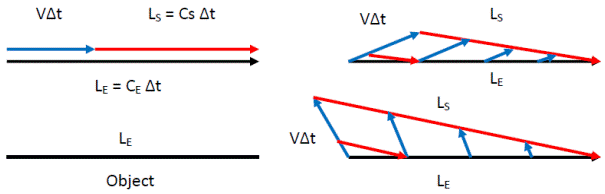Fig. 6. The Visions of Light (red lines) observed by the observers at different moving speeds and directions (blue lines) with respect to the Vision of Light (black line) observed by the observer at ground.

LE = V∆t + LS

LS/LE = (LE – V∆t)/LE

LS /LE = (CE-V) ∆t/CE∆t = (CE-V)/CE

LS = (1-V/CE) LE

Ls < LE

Depending on the moving speed and direction of the observer, the length of object, which is equal to the Vision of Light, can either shrink or expand. In another word, both Length Contraction and Length Expansion can happen subject to the moving speeds and directions of the observers with respect to the object.

10. Time

Time is the measurement of the cycles of a fundamental process in the Universe such as the electronic transition in atomic clock. Each event takes exactly the same amount of cycles (Time) from beginning to end (Experience) no matter where the event takes place. However, the period of the clock can be different from one location to the other. A place with longer period of clock will have slower Time. For example, a 3000 – cycles event or life (Time) can be completed sooner on Earth than Saturn, because the clock on Earth is faster (shorter period) than that on Saturn due to the less gravitational field on Earth.

11. Velocity Time Dilation

In Einstein’s Special Relativity (Ref. 3), it is assumed that light speed in vacuum is always a constant no matter where the light sources and observers are. As a consequence, Time on a moving object runs slower than that is stationary to observer which is known as Velocity Time Dilation (Ref. 9).

Because that the light speeds in vacuum is not a constant, Einstein’s Special Relativity is incorrect, so is that the Velocity Time Dilation. In facts, there are three conflicts and mistakes in the derivation of Velocity Time Dilation (Fig. 7):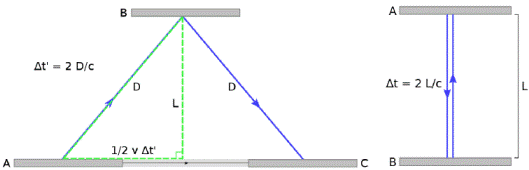Fig. 7. Moving light clock and Velocity Time Dilation Theory.

1.   Speed of Light: The assumptions that light speeds observed by both ground observer and moving observer at light source are equal which against the principles of Vision of Light (Fig. 1).

2.   Direction of Light: What if the light clock is placed in a tilted angel or horizontal direction instead of perpendicular direction with respect to the moving direction? Do we still have the same Velocity Time Dilation? The answer is no, because (∆t’C/2)2 = (∆tC/2)2 + (V ∆t’/2)2 and ∆t’ = (1 – V2/C2)-1/2 ∆t are no longer true for a tilted angel or horizontal direction.

3.   Twin Paradox: Motion is relative, whatever the motions that twin brothers experienced in their own time systems, either in spaceship or on Earth, are identical except in opposite directions. Slower time and younger life can be claimed by both brothers which conflicts to the common principles of logic. Therefore Twin Paradox (Ref. 10) proves that Velocity Time Dilation is wrong and can never exist.

12. Gravitational Time Dilation

Time can be influenced by gravitational field as claimed by Einstein’s General Relativity (Ref. 3 & Ref. 11), clocks that are far from massive bodies or at higher gravitational potentials run more quickly, and clocks close to massive bodies or at lower gravitational potentials run more slowly. This phenomenon is known as Gravitational Time Dilation (Ref. 12).

Time is the measurement of the cycles of a fundamental process in the Universe. Since Wu’s Particle (Yangton and Yington Circulation Pair) is the building block of all matters, its circulation cycle is the nature clock build in all substances or systems.

It is assumed that large gravitation field will cause the increase of the period and decrease of the frequency of Yangton and Yington circulation and thus to slow down the cycles of the clock – "Time". This agrees to Einstein’s General Relativity.

13. Conclusion

The second postulate of Einstein’s Special Relativity that "The speed of light in a vacuum is the same for all observers, regardless of their relative motion or the motion of the light source" is incorrect. Light Speed in vacuum, instead of being a constant, it changes with those observers moving at different speeds and directions with respect to light origin.

Because that the photon emission only requires a fixed amount of Force of Separation, and also it is a Non-Inertia Transformation predicted by Yangton and Yington theory and proved by Red Shift phenomenon, light travels in vacuum with a constant Absolute Light Speed C in a straight direction can be observed by the observers at light origins and those in stationary with light origins in Absolute Space System.

Since different light speeds in vacuum can be observed by observers other than those at light origins and in stationary with light origins in Absolute Space System, therefore Velocity Time Dilation derived from Einstein’s Special Relativity is not true and could never exist.

Absolute Space System, Vision of Light and Non-Inertia Transformation are introduced to explain the relationships between Space, Time and Special Relativity. Doppler Effect, Blue Shift and Red shift are due to the Non-Inertia Transformation of light emission. Length Contraction is caused by the difference of Visions of Light instead of Velocity Time Dilation.

In an Inertia System, for a given light beam, the same light speeds in vacuum can be observed by all the observers. Furthermore, Michelson - Morley Experiment proves that for two split light beams traveling, the same light speeds can also be observed.

Time is the measurement of the cycles of a fundamental process in the Universe. Both time and light speed at large gravitational field have relatively slower rates. This may be caused by the longer period and lower frequency of Yangton and Yington circulation due to the large gravitational field. This agrees well with Gravitational Time Dilation in Einstein’s General Relativity.

References

1. Edward T. H. Wu, "Yangton and Yington – A Hypothetical Theory of Everything", Science Journal of Physics, Volume 2015, Article ID sjp-242, 6 Pages, 2015, doi: 10.7237/sjp/242.
2. Edward T. H. Wu. Subatomic Particle Structures and Unified Field Theory Based on Yangton and Yington Hypothetical Theory. American Journal of Modern Physics. Vol. 4, No. 4, 2015, pp. 165-171. doi: 10.11648/j.ajmp.20150404.13.
3. Einstein A. (1916), Relativity: The Special and General Theory (Translation 1920), New York: H. Holt and Company.
4. Alec Eden The search for Christian Doppler, Springer-Verlag, Wien 1992. Contains a facsimile edition with an English translation.
5. O'Connor, John J.; Robertson, Edmund F. (1998). "Christian Andreas Doppler". MacTutor History of Mathematics archive. University of St Andrews.
6. Kuhn, Karl F.; Theo Koupelis (2004). In Quest of the Universe. Jones & Bartlett Publishers. pp. 122–3. ISBN 0-7637-0810-0.
7. FitzGerald, George Francis (1889),"The Ether and the Earth's Atmosphere", Science 13 (328):390, Bibcode:1889Sci....13.390F, doi:10.1126/science.ns13.328.390,PMID 17819387.
8. Lorentz, Hendrik Antoon (1892),"The Relative Motion of the Earth and the Aether", Zittingsverlag Akad. V. Wet. 1: 74–79.
9. Einstein, Albert (1920). "On the Idea of Time in Physics". Relativity: The Special and General Theory. Henri Holt. ISBN 1-58734-092-5. And also in sections 9–12.
11. https://en.wikipedia.org/wiki/General_relativity.
12. A. Einstein, "Über das Relativitätsprinzip und die aus demselben gezogenen Folgerungen", Jahrbuch der Radioaktivität und Elektronik 4, 411–462 (1907); English translation, in "On the relativity principle and the conclusions drawn from it", in "The Collected Papers", v.2, 433–484 (1989); also in H M Schwartz, "Einstein's comprehensive 1907 essay on relativity, part I", American Journal of Physics vol.45,no.6 (1977) pp.512–517; Part II in American Journal of Physics vol.45 no.9 (1977), pp.811–817; Part III in American Journal of Physics vol.45 no.10 (1977), pp.899–902, see parts I, II and III.

 Contents 1. 2. 3. 4. 5. 5.1. 5.2. 5.3. 5.4. 5.5. 6. 7. 8. 9. 10. 11. 12. 13.
Article ToolsAbstractPDF(1470K)